Question

# on Help A sample of 51 values is taken from population that is normally distributed with...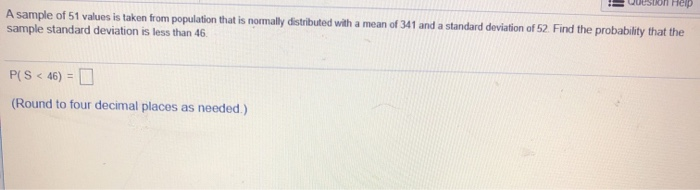on Help A sample of 51 values is taken from population that is normally distributed with a mean of 341 and a standard deviation of 52. Find the probability that the sample standard deviation is less than 46. PCS < 46) = 0 (Round to four decimal places as needed.)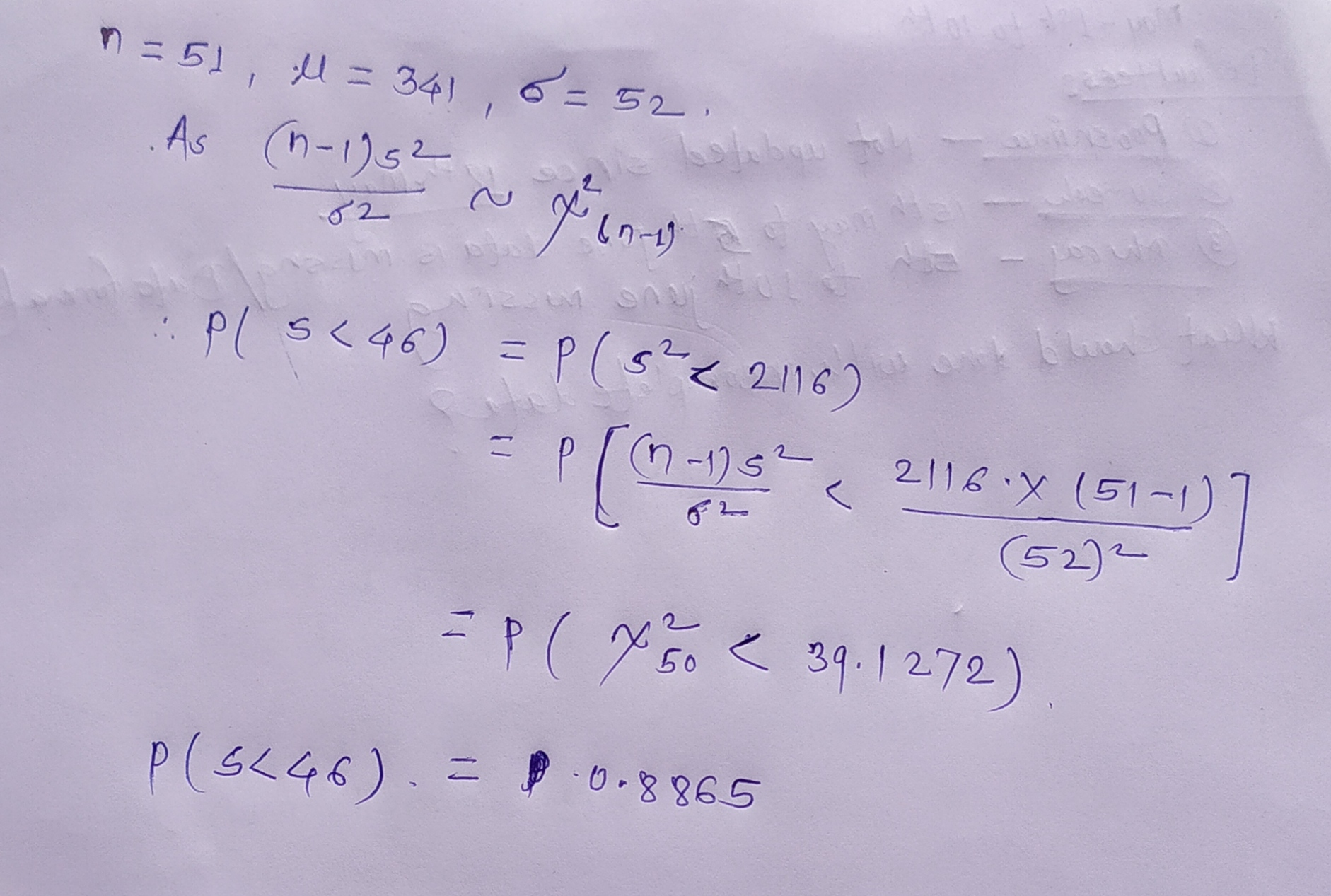I have given my best to solve your problem. Please like the answer if you are satisfied with it. ?

#### Earn Coins

Coins can be redeemed for fabulous gifts.

Similar Homework Help Questions
• ### A normally distributed population has a mean of 475 and a standard deviation of 48. a....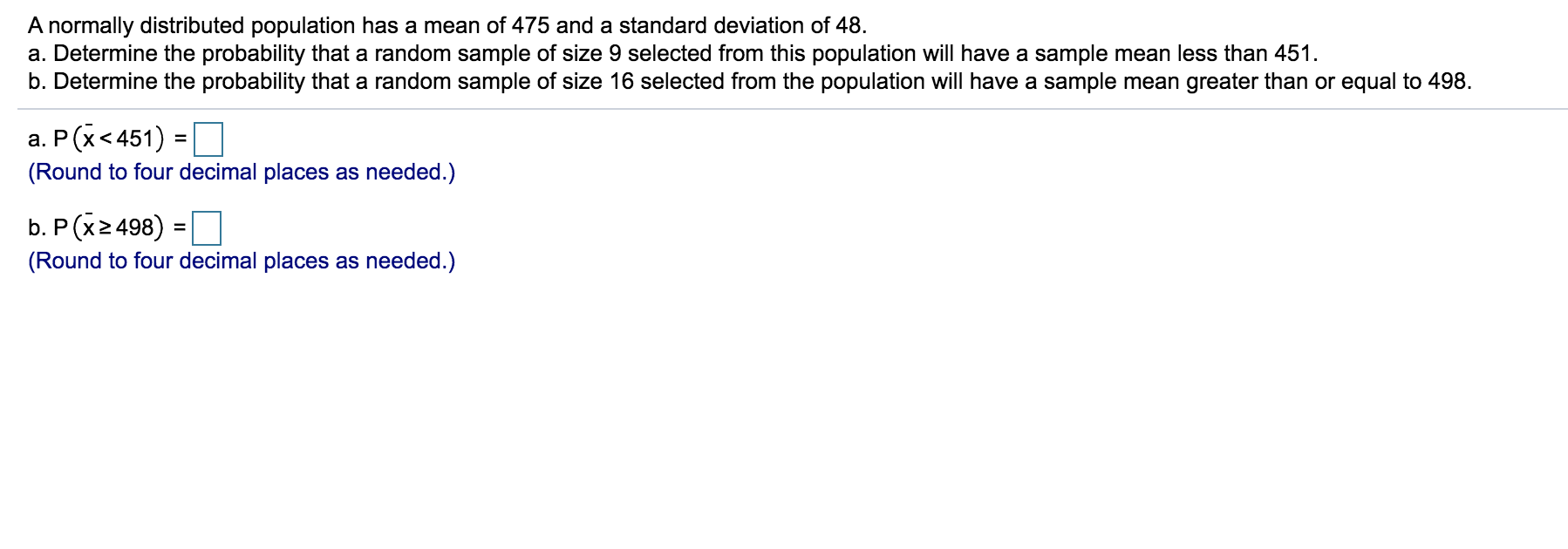A normally distributed population has a mean of 475 and a standard deviation of 48. a. Determine the probability that a random sample of size 9 selected from this population will have a sample mean less than 451. b. Determine the probability that a random sample of size 16 selected from the population will have a sample mean greater than or equal to 498. a. P(X<451) = (Round to four decimal places as needed.) b. P(X2498) = 1 (Round to...

• ### A random sample of 18 observations taken from a population that is normally distributed produced a...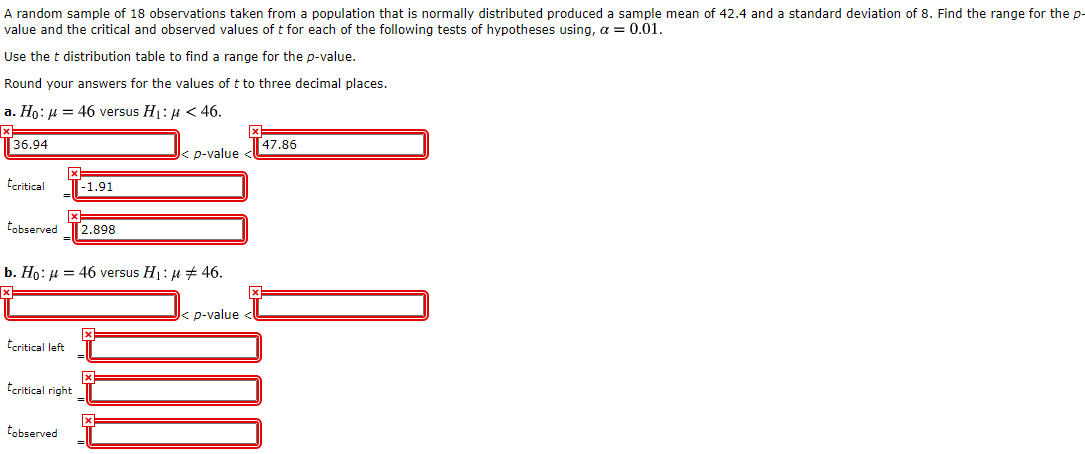A random sample of 18 observations taken from a population that is normally distributed produced a sample mean of 42.4 and a standard deviation of 8. Find the range for the p- value and the critical and observed values of t for each of the following tests of hypotheses using, a = 0.01. Use the t distribution table to find a range for the p-value. Round your answers for the values of t to three decimal places. a. Ho:u= 46...

• ### A random sample of size 12 was taken from a normally distributed population with a population...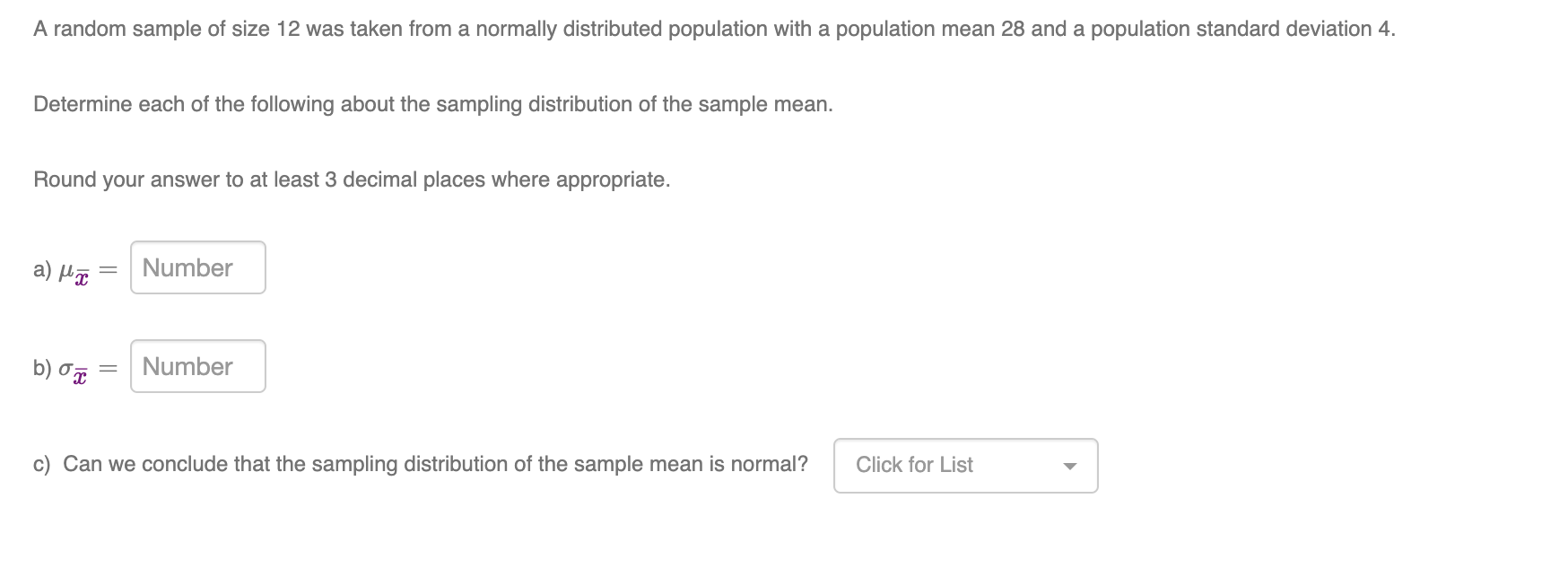A random sample of size 12 was taken from a normally distributed population with a population mean 28 and a population standard deviation 4. Determine each of the following about the sampling distribution of the sample mean. Round your answer to at least 3 decimal places where appropriate. a) un Number b) 0 = Number c) Can we conclude that the sampling distribution of the sample mean is normal? Click for List

• ### Arandom sample of 21 observations taken from a population that is normally distributed produced a sample...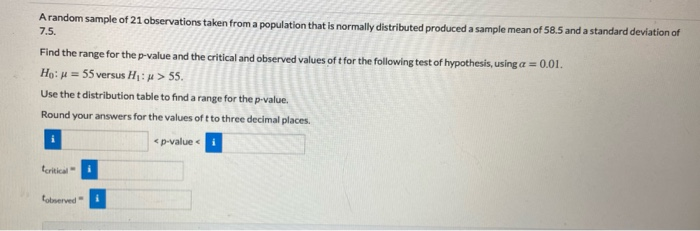Arandom sample of 21 observations taken from a population that is normally distributed produced a sample mean of 58.5 and a standard deviation of 7.5. Find the range for the p-value and the critical and observed values of tfor the following test of hypothesis, using a = 0.01. Ho: = 55 versus H: > 55. Use the t distribution table to find a range for the p-value Round your answers for the values oft to three decimal places. <p-value torta...

• ### A random sample of size 15 was taken from a normally distributed population with a population...

A random sample of size 15 was taken from a normally distributed population with a population mean 26 and a population standard deviation 4. Determine each of the following about the sampling distribution of the sample mean. Round your answer to at least 3 decimal places where appropriate. a) μx_=    b) σx_=    c)  Can we conclude that the sampling distribution of the sample mean is normal?

• ### Suppose a random sample of size 17 was taken from a normally distributed population, and the...

Suppose a random sample of size 17 was taken from a normally distributed population, and the sample standard deviation was calculated to be s = 5.0. a) Calculate the margin of error for a 95% confidence interval for the population mean. Round your response to at least 3 decimal places.     b) Calculate the margin of error for a 90% confidence interval for the population mean. Round your response to at least 3 decimal places.

• ### A random experiment involves drawing a sample of 12 data values from a normally distributed population....

A random experiment involves drawing a sample of 12 data values from a normally distributed population. 20 29 39 42 43 45 45 46 47 54 55 59 Calculate the z-score of the median of the data set. z =  (Round to 3 decimal places) Hint: You will need the mean and standard deviation first. Round your mean and standard deviation calculations to 5 decimal places.

• ### 5.4.1 Question Help A population has a mean = 141 and a standard deviation o =...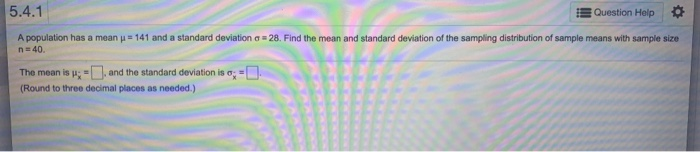5.4.1 Question Help A population has a mean = 141 and a standard deviation o = 28. Find the mean and standard deviation of the sampling distribution of sample means with sample size n = 40. The mean is :-), and the standard deviation is 0;=0 (Round to three decimal places as needed.) 5.4.2 Question Help A population has a meanu - 74 and a standard deviation = 8. Find the mean and standard deviation of a sampling distribution of...

• ### Please give explanation Help Save & A random sample is drawn from a normally distributed population...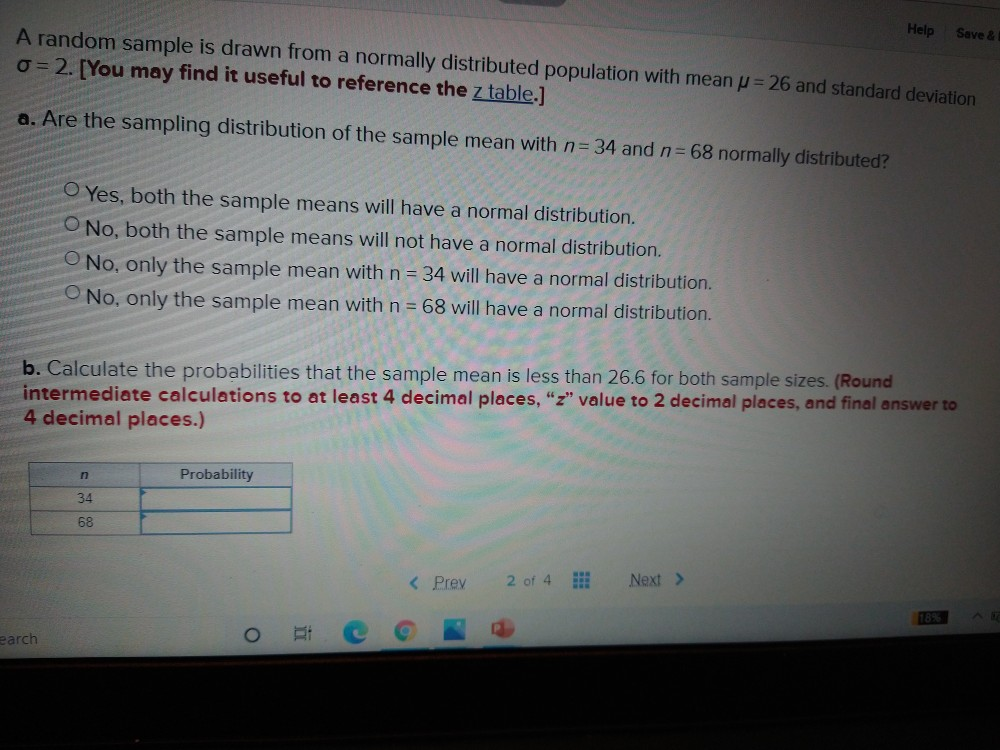Please give explanation Help Save & A random sample is drawn from a normally distributed population with mean u = 26 and standard deviation o=2. [You may find it useful to reference the z table.] a. Are the sampling distribution of the sample mean with n= 34 and n=68 normally distributed? O Yes, both the sample means will have a normal distribution. O No, both the sample means will not have a normal distribution. No, only the sample mean with...

• ### Question 1: A random sample of 22 observations taken from a population that is normally distributed...

Question 1: A random sample of 22 observations taken from a population that is normally distributed produced a sample mean of 58.5 and a standard deviation of 7.5. Find the range for the p-value and the critical and observed values of t for the following test of hypothesis, using α = 0.01 . H 0 :   μ = 55 versus H 1 :   μ > 55 . Use the t distribution table to find a range for the p-value. Question 2: A...